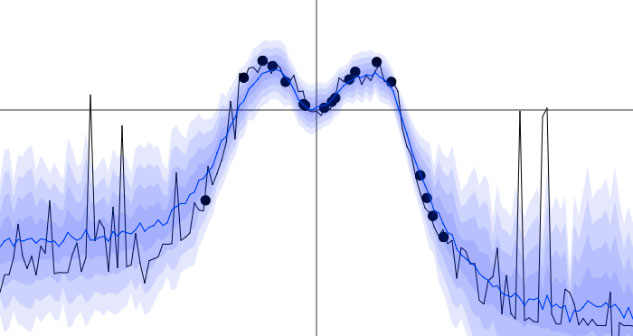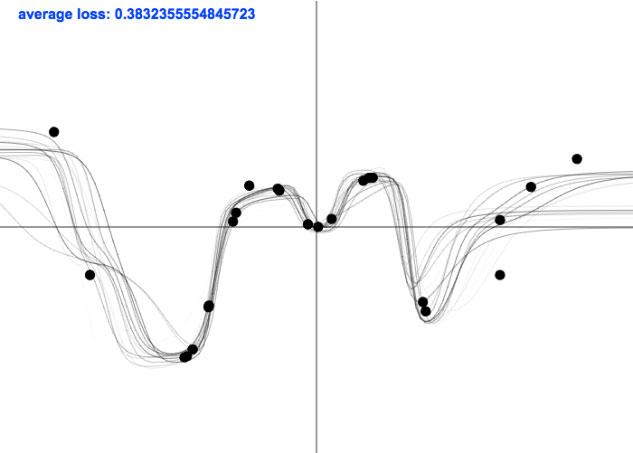## Uncertainty in Deep Learning (PhD Thesis)

October 13th, 2016 (Updated: June 4th, 2017)##### Function draws from a dropout neural network. This new visualisation technique depicts the distribution over functions rather than the predictive distribution (see demo below).
So I finally submitted my PhD thesis (given below). In it I organised the already published results on how to obtain uncertainty in deep learning, and collected lots of bits and pieces of new research I had lying around (which I hadn't had the time to publish yet). The questions I got about the work over the past year were a great help in guiding my writing, with the greatest influence on my writing, I reckon, being the work of Professor Sir David MacKay (and his thesis specifically). Weirdly enough, I would consider David's writing style to be the equivalent of modern blogging, and would highly recommend reading his thesis. I attempted to follow David's writing style in my own writing, explaining topics through examples and remarks, resulting in what almost looks like a 120 pages long blog post. So hopefully it can now be seen as a more complete body of work, accessible to as large an audience as possible, and also acting as an introduction to the field of what people refer to today as Bayesian Deep Learning. One of the interesting results which I will demonstrate below touches on uncertainty visualisation in Bayesian neural networks. It's something that almost looks trivial, yet it has gone unnoticed for quite some time! But before that, I'll review quickly some of the new bits and pieces in the thesis for people already familiar with the work. For others I would suggest starting with the introduction: The Importance of Knowing What We Don't Know.

### Thesis: Uncertainty in Deep Learning

Some of the work in the thesis was previously presented in [Gal, 2015; Gal and Ghahramani, 2015a,b,c,d; Gal et al., 2016], but the thesis contains many new pieces of work as well. The most notable of these are

1. some discussions: a discussion of AI safety and model uncertainty (§1.3), a historical survey of Bayesian neural networks (§2.2),
2. some theoretical analysis: a theoretical analysis of the variance of the re-parametrisation trick and other Monte Carlo estimators used in variational inference (the re-parametrisation trick is not a universal variance reduction technique! §3.1.1§3.1.2), a survey of measures of uncertainty in classification tasks (§3.3.1),
3. some empirical results: an empirical analysis of different Bayesian neural network priors (§4.1) and posteriors with various approximating distributions (§4.2), new quantitative results comparing dropout to existing techniques (§4.3), tools for heteroscedastic model uncertainty in Bayesian neural networks (§4.6),
4. some applications: a survey of recent applications in language, biology, medicine, and computer vision making use of the tools presented in this thesis (§5.1), new applications in active learning with image data (§5.2),
5. and more theoretical results: a discussion of what determines what our model uncertainty looks like (§6.1§6.2), an analytical analysis of the dropout approximating distribution in Bayesian linear regression (§6.3), an analysis of ELBO-test log likelihood correlation (§6.4), discrete prior models (§6.5), an interpretation of dropout as a proxy posterior in spike and slab prior models (§6.6, relating dropout to works by MacKay, Nowlan, and Hinton from 1992), as well as a procedure to optimise the dropout probabilities based on the variational interpretation to separate the different sources of uncertainty (§6.7).
The thesis can be obtained as a Single PDF (9.1M), or as individual chapters (since the single file is fairly large):
• Contents (PDF, 36K)
• Chapter 1: The Importance of Knowing What We Don't Know (PDF, 393K)
• Chapter 2: The Language of Uncertainty (PDF, 136K)
• Chapter 3: Bayesian Deep Learning (PDF, 302K)
• Chapter 4: Uncertainty Quality (PDF, 2.9M)
• Chapter 5: Applications (PDF, 648K)
• Chapter 6: Deep Insights (PDF, 939K)
• Chapter 7: Future Research (PDF, 28K)
• Bibliography (PDF, 72K)
• Appendix A: KL condition (PDF, 71K)
• Appendix B: Figures (PDF, 2M)
• Appendix C: Spike and slab prior KL (PDF, 28K)
I would appreciate it if you could cite this thesis (BiBTex) if you intend to use any of the new results.

One of the nice practical new results in section §4.1 for example affects function visualisation. It's a minor change that has gone unnoticed until now, but which is significant in understanding our functions.

### Function visualisation

There are two factors at play when visualising uncertainty in dropout Bayesian neural networks: the dropout masks and the dropout probability of the first layer. Uncertainty depictions in my previous blog posts drew new dropout masks for each test point—which is equivalent to drawing a new prediction from the predictive distribution for each test point $-2 \leq \x \leq 2$. More specifically, for each test point $\x_i$ we drew a set of network parameters from the dropout approximate posterior $\boh_{i} \sim q_\theta(\bo)$, and conditioned on these parameters we drew a prediction from the likelihood $\y_i \sim p(\y | \x_i, \boh_{i})$. Since the predictive distribution has \begin{align*} p&(\y_i | \x_i, \X_\train, \Y_\train) \\ &= \int p(\y_i | \x_i, \bo) p(\bo | \X_\train, \Y_\train) \text{d} \bo \\ &\approx \int p(\y_i | \x_i, \bo) q_\theta(\bo) \text{d} \bo \\ &=: q_\theta(\y_i | \x_i) \end{align*} we have that $\y_i$ is a draw from an approximation to the predictive distribution.##### Figure A: In black is a draw from the predictive distribution of a dropout neural network $\yh \sim q_\theta(\y | \x)$ for each test point $-2 \leq \x \leq 2$, compared to the function draws in figure B.
This process is equivalent to drawing a new function for each test point, which results in extremely erratic depictions that have peaks at different locations (seen in figure A taken from the previous blog post). Drawing a new function for each test point makes no difference if all we care about is obtaining the predictive mean and predictive variance (actually, for these two quantities this process is preferable to the one I will describe below), but this process does not result in draws from the induced distribution over functions. This is because different network parameters correspond to different functions, and a distribution over the network parameters therefore induces a distribution over functions. Under a Bayesian interpretation, we identify a draw $\boh$ from the posterior over network parameters $q_\theta(\bo)$ with a single function draw. To get a draw from our induced posterior over functions, we would need to sample a single network for all test points then, rather than sample a particular prediction for each test point.##### Figure B: Each solid black line is a function drawn from a dropout neural network posterior over functions, induced by a draw from the approximate posterior over the weights $\boh \sim q_\theta(\bo)$.
To visualise our predictive distribution in a more appealing way we could draw a single network for the entire test set. With dropout, this can be done by drawing a single set of masks to be used with all test points. Our induced functions look very different now (seen in figure B, and with a demo below). In the new visualisation the functions are smooth, even though they are drawn from a dropout approximating distribution (which randomly sets whole rows of the weight matrix to zero). Note that to calculate predictive mean and predictive variance, using different masks is actually preferable since it results in lower variance estimators.

Another important factor affecting visualisation is the dropout probability of the first layer. In the previous posts we depicted scalar functions and set all dropout probabilities to $0.1$. As a result, with probability $0.1$, the sampled functions from the posterior would be identically zero. This is because a zero draw from the Bernoulli distribution in the first layer together with a scalar input leads the model to completely drop its input (explaining the points where the function touches the $x$-axis in figure A). This is a behaviour we might not believe the posterior should exhibit (especially when a single set of masks is drawn for the entire test set), and could change this by setting a different probability for the first layer. Setting $p_1 = 0$ for example is identical to placing a delta approximating distribution over the first weight layer.

In the demo below we use $p_1 = 0$, and depict draws from the approximate predictive distribution evaluated on the entire test set $q_{\theta_i}(\Y | \X, \boh_i)$ ($\boh_i \sim q_{\theta_i}(\bo)$, function draws), as the variational parameters $\theta_i$ change and adapt to minimise the divergence to the true posterior (with old samples disappearing after 20 optimisation steps). You can change the function the data is drawn from (with two functions, one from the last blog post and one from the appendix in this paper), and the model used (a homoscedastic model or a heteroscedastic model, see section §4.6 in the thesis for example or this blog post).

Browser not supported for Canvas. Get a real browser.

## Acknowledgements

To finish this blog post I would like to thank the people that helped through comments and discussions during the writing of the various papers composing the thesis above. I would like to thank (in alphabetical order) Christof Angermueller, Yoshua Bengio, Phil Blunsom, Yutian Chen, Roger Frigola, Shane Gu, Alex Kendall, Yingzhen Li, Rowan McAllister, Carl Rasmussen, Ilya Sutskever, Gabriel Synnaeve, Nilesh Tripuraneni, Richard Turner, Oriol Vinyals, Adrian Weller, Mark van der Wilk, Yan Wu, and many other reviewers for their helpful comments and discussions. I would further like to thank my collaborators Rowan McAllister, Carl Rasmussen, Richard Turner, Mark van der Wilk, and my supervisor Zoubin Ghahramani.

Lastly, I would like to thank Google for supporting three years of my PhD with the Google European Doctoral Fellowship in Machine Learning, and Qualcomm for supporting my fourth year with the Qualcomm Innovation Fellowship.

PS. there might be some easter eggs hidden in the introduction :)

## Contact me#### Email

yarin@cs.ox.ac.uk#### Post

Computer Science Department
University of Oxford
Oxford, OX1 3QD
United Kingdom